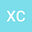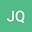The number of Dirac-weighted eigenvalues of Sturm-Liouville equations with integrable potentials and an application to inverse problems
••• Xiao Chen,
• Jiangang Qi
Xiao Chen
Shandong University at Weihai

Corresponding Author:chenxiao@sdu.edu.cn

Author ProfileJiangang Qi
Shandong University at Weihai
Author Profile## Abstract

In this paper, we further Meirong Zhang, et al.’s work by computing the number of weighted eigenvalues for Sturm-Liouville equations, equipped with general integrable potentials and Dirac weights, under Dirichlet boundary condition. We show that, for a Sturm-Liouville equation with a general integrable potential, if its weight is a positive linear combination of $n$ Dirac Delta functions, then it has at most $n$ (may be less than $n$, or even be $0$) distinct real Dirichlet eigenvalues, or every complex number is a Dirichlet eigenvalue; in particular, under some sharp condition, the number of Dirichlet eigenvalues is exactly $n$. Our main method is to introduce the concepts of characteristics matrix and characteristics polynomial for Sturm-Liouville problem with Dirac weights, and put forward a general and direct algorithm used for computing eigenvalues. As an application, a class of inverse Dirichelt problems for Sturm-Liouville equations involving single Dirac distribution weights is studied.
29 Dec 2020Submitted to Mathematical Methods in the Applied Sciences
30 Dec 2020Assigned to Editor
30 Dec 2020Submission Checks Completed
10 Jan 2021Reviewer(s) Assigned
04 May 2021Review(s) Completed, Editorial Evaluation Pending
05 May 2021Editorial Decision: Accept
30 Sep 2021Published in Mathematical Methods in the Applied Sciences volume 44 issue 14 on pages 11702-11719. 10.1002/mma.7547